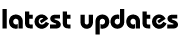Ganit Mantra Series : Complex Number-1

Introduction:

Complex numbers is very important topic for sciences and engineering students and is a powerful tool to solve many mathematical problems. However, the concept of numbers and knowledge of this portion of mathematics for the students is not very much clear , Therefore, this section of complex numbers is primarily focused on fundamental concept , where they will be able to understand the basic nature of complex number and their actual significance . Thus, statements and concepts  that will be useful for students are presented here.

You must all be familiar by now with the various number systems, starting from the natural numbers, to the real numbers.

• Natural Numbers N         : 1, 2, 3…….
• Whole numbers   W     : 0, 1, 2, 3…….
• Integers    Z         : – 3, –2, –1, 0, 1, 2, 3 ……..

Now ,  Here we will discuss about complex number which will have two part real and imaginary. e.g Z = x+iy ,  Where x is called real part and y is called imaginary part .

We will discuss this topic in several segment 1-6 .To read the first one click on the following link :My Great Web page